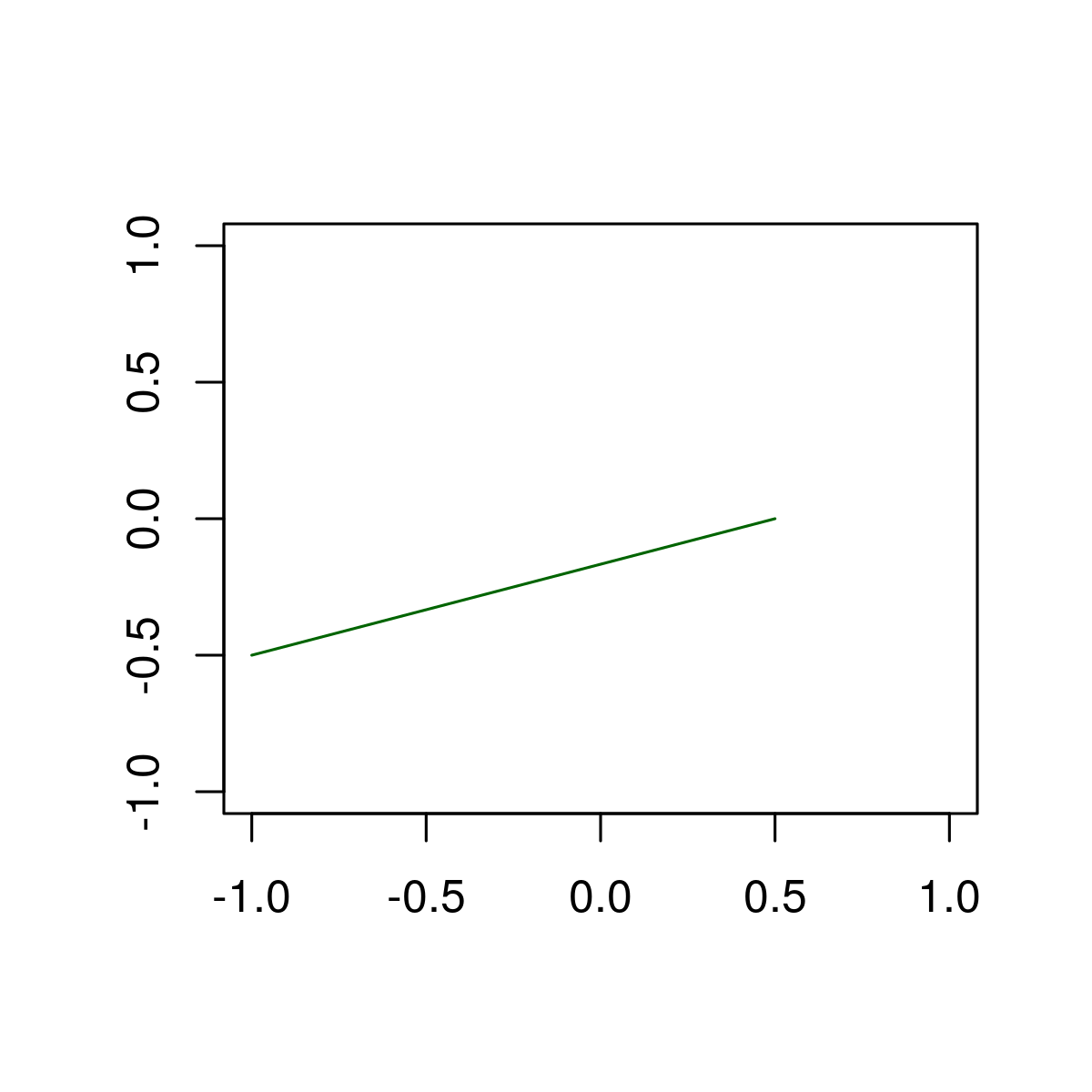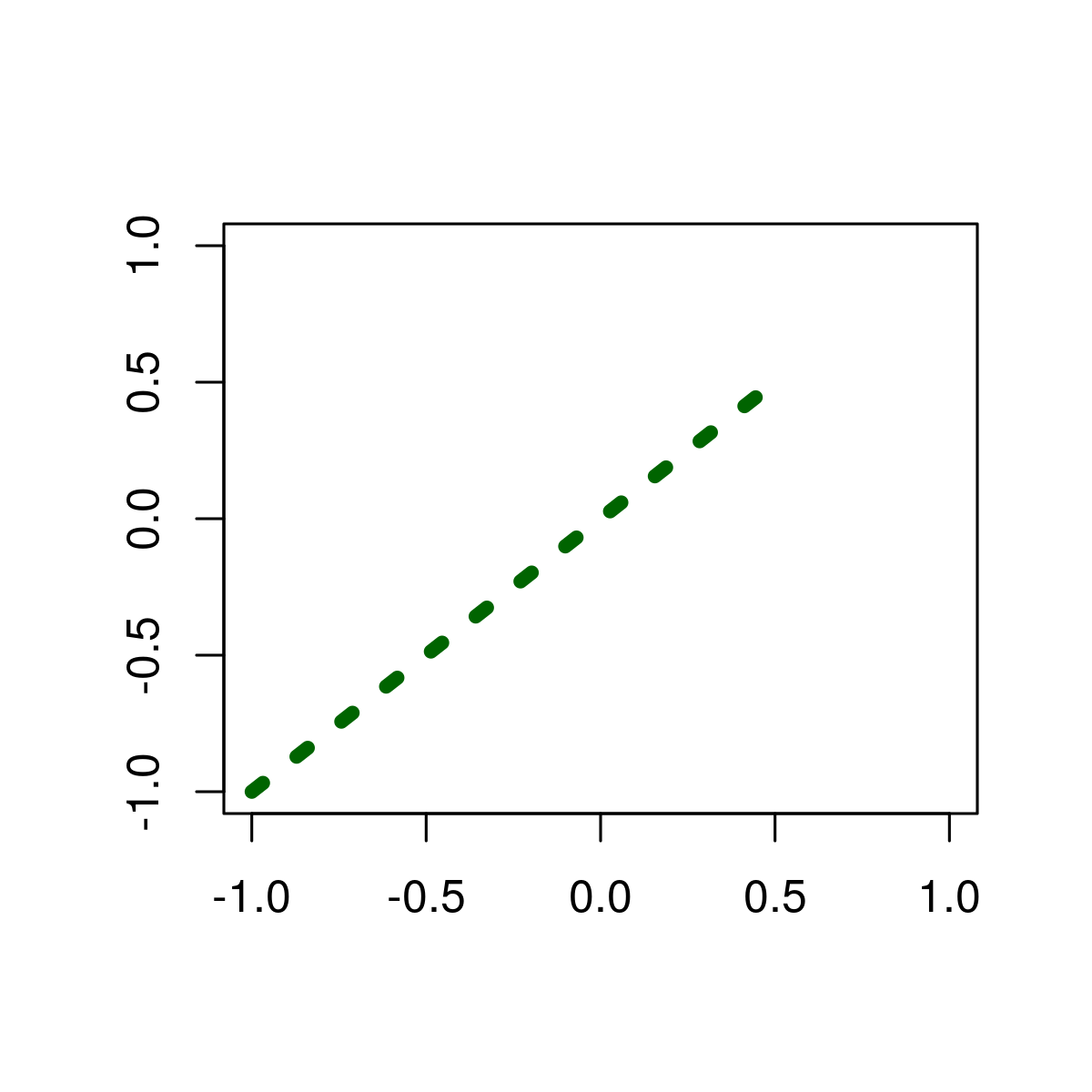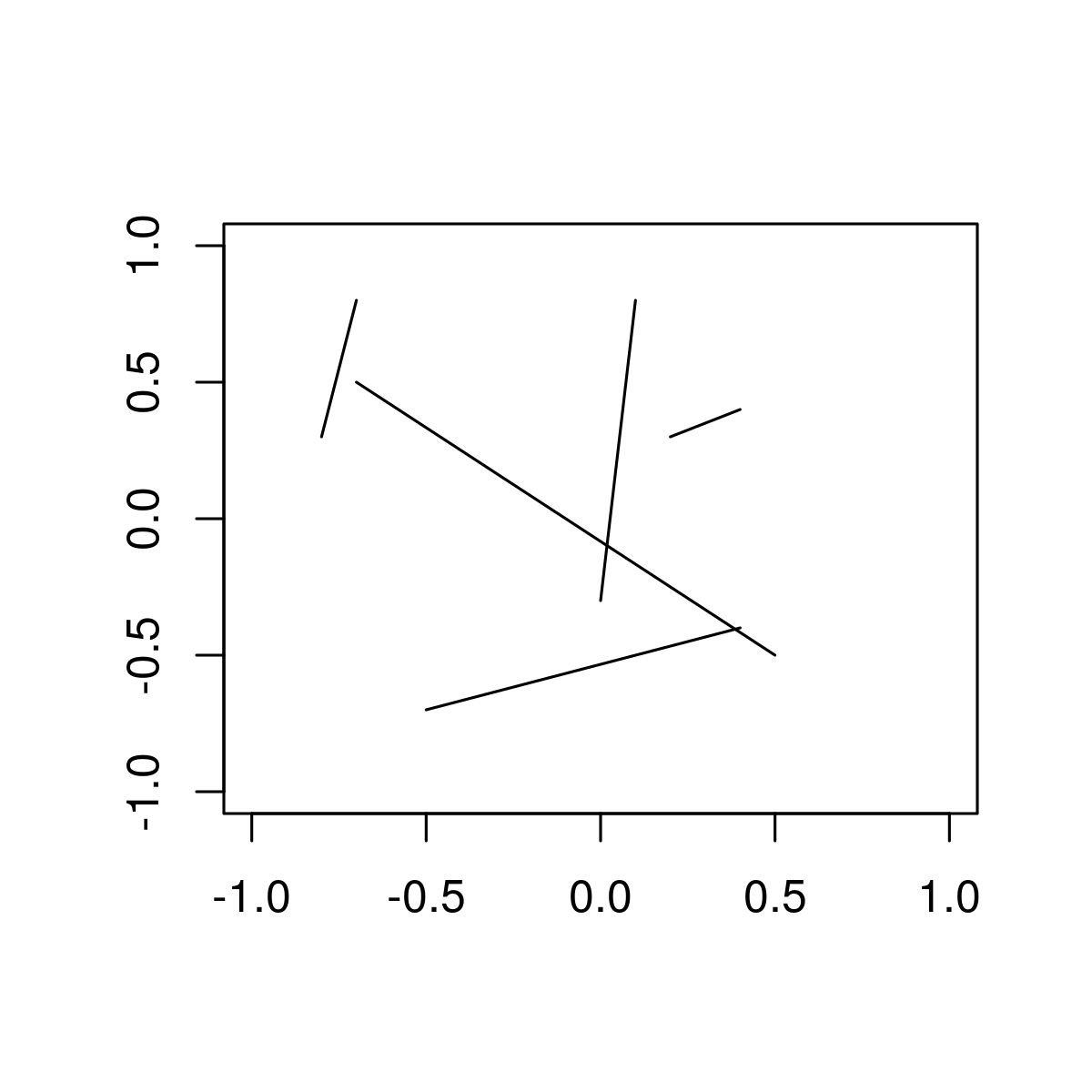Related Articles

# Draw Line Segments between Particular Points in R Programming – segments() Function

• Last Updated : 19 Jun, 2020

`segment()` function in R Language is used to draw a line segment between to particular points.

Syntax: segments(x0, y0, x1, y1)

Parameters:
x, y: coordinates to draw a line segment between provided points.

Returns: a line segment between given points

Example 1: To draw a single line segment

 `# Create empty example plot``plot``(0, 0, col = ``"white"``, xlab = ``""``, ylab = ``""``)`` ` `# Draw one line``segments``(x0 = - 1, y0 = - 0.5, x1 = 0.5, y1 = 0, col = ``"darkgreen"``) `

Output:Here, x0 & y0 are starting points of the line segment and x1 & y1 are ending points of line segment .

Example 2: Modifying  color, thickness & line type

 `# Create empty example plot``plot``(0, 0, col = ``"white"``, xlab = ``""``, ylab = ``""``)`` ` `# Draw one line as in Example 1``segments``(x0 = - 1, y0 = - 1, x1 = 0.5, y1 = 0.5,``          ` `         ``# Color of line``         ``col = ``"darkgreen"``,      ``          ` `         ``# Thickness of line``         ``lwd = 5,                ``          ` `         ``# Line type``         ``lty = ``"dotted"``)                     `

Output:Example 3: Draw multiple line segments to R Plot.

 `# Create empty example plot``plot``(0, 0, col = ``"white"``, xlab = ``""``, ylab = ``""``)       `` ` `# Create data frame with line-values``multiple_segments <- ``data.frame``(x0 = ``c``(0.1, 0.2, - 0.7, 0.4, - 0.8),   ``                       ``y0 = ``c``(0.8, 0.3, 0.5, - 0.4, 0.3),``                       ``x1 = ``c``(0, 0.4, 0.5, - 0.5, - 0.7),``                       ``y1 = ``c``(- 0.3, 0.4, - 0.5, - 0.7, 0.8))``                        ` ` ``# Draw multiple lines                       `` ``segments``(x0 = multiple_segments\$x0,                   ``         ``y0 = multiple_segments\$y0,``         ``x1 = multiple_segments\$x1,``         ``y1 = multiple_segments\$y1)`

Output:My Personal Notes arrow_drop_up